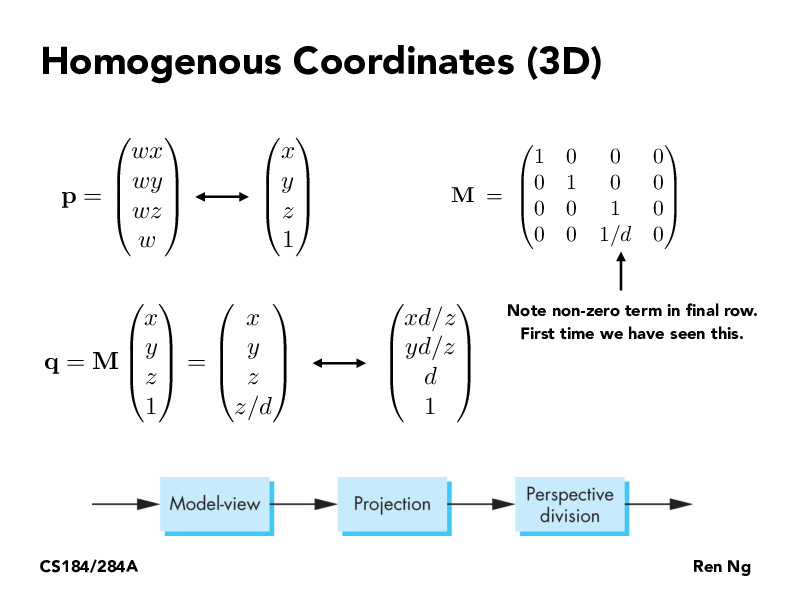Lecture 4: Transforms (83)evan1997123

Although the M looks a bit weird and there's just so much transformation matrices that are being shown to us, I think that is cool that we are able to just multiply all of the M's together to create one single Transformation Matrix M that will do so many different operations into one. Then, we could just hold onto those and never have to compute them. I like how this has been createdNicoDeshler

I still don't think I understand how M is working here? Isn't the intended transformation for perspective projection to take the vector (x,y,z) in 3D space and map it to (xd/z, yd/z, d) as shown by the 'similar triangles' argument on the previous slide? Wouldn't this then simply be a diagonal matrix with the following factors in the diagonal entries (d/z,d/z,/d)? I think I am missing why we would need homogenous coordinates for this transformation. Thanks!dangeng184

@Nico (hi, good to see you here). I think if you did it your way you would need a different matrix for each point you transform (because the z is variable). Doing it in homogenous coordinates allows you to use just a single transformation matrix for all the points.NicoDeshler

Ah I see your point. Thanks Daniel! Just to make sure I understand correctly, we would still use the z-coordinate of every point to multiply the final vector $(x, y, z, z/d)$ by $d/z$, we just wouldn't instantiate a new matrix for every point?

You must be enrolled in the course to comment